# Overview

This article will explain the Model Groups feature in ONE DATA. It offers the possibility to create an arbitrary number of Models within the available Python Script Processors (linked under Related Articles).

This is a feature for advanced ONE DATA users, so to use it efficiently, you should be familiar with Python, the usage of the respective Python Processors and have basic knowledge of the ONE DATA Python Framework and Models.

The advantage of this new feature is, that you can define the amount of needed Models at runtime and you don't have to specify the name of every Model in advance, which can be very useful when generating Models dynamically.

# Configuration Options for Python Processors

The Model Groups feature introduces some new configuration options for the ONE DATA Python Processors, which will be explained in this section.

## Save One or More Model Groups With Assigned Models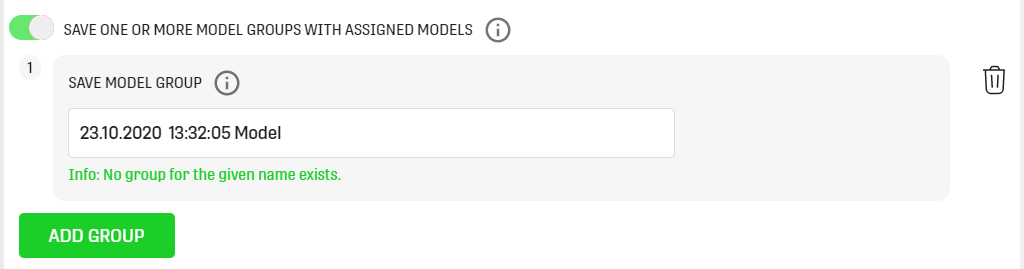With this option, you can save a Model Group created by Python within ONE DATA. All Model Groups added in the Python script must be configured in here, otherwise they will not be saved to the ONE DATA environment. To save a Model assigned to a Model Group stored in a variable Model under the name "my_model" and the Model Group name \"my_model_group\" use the following statement in the script:

`od_output.add_model("my_model", model, "my_model_group")`

`Note that Models or Model Groups need to have a unique name within a Domain in ONE DATA.`

## Load One or More Model Groups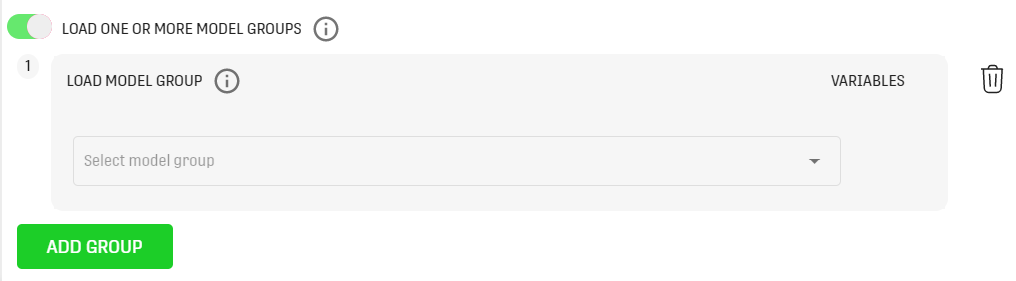By using this option, it is possible to load Model Groups for Python execution. Models of all loaded Model Groups will be accessible in the Python code in the dictionary: od_models

To load a Model named "my_model" and store it in a variable Model use the following statement:

`model = od_models["my_model"]`

# View Existing Model Groups

At the moment, unfortunately, there is no overview in ONE DATA that lists the existing Model Groups you have access to. As a workaround, you can open the configuration of any Python Processor and check the dropdown values of the "Load One or More Model Groups" option. There, all available Model Groups are listed.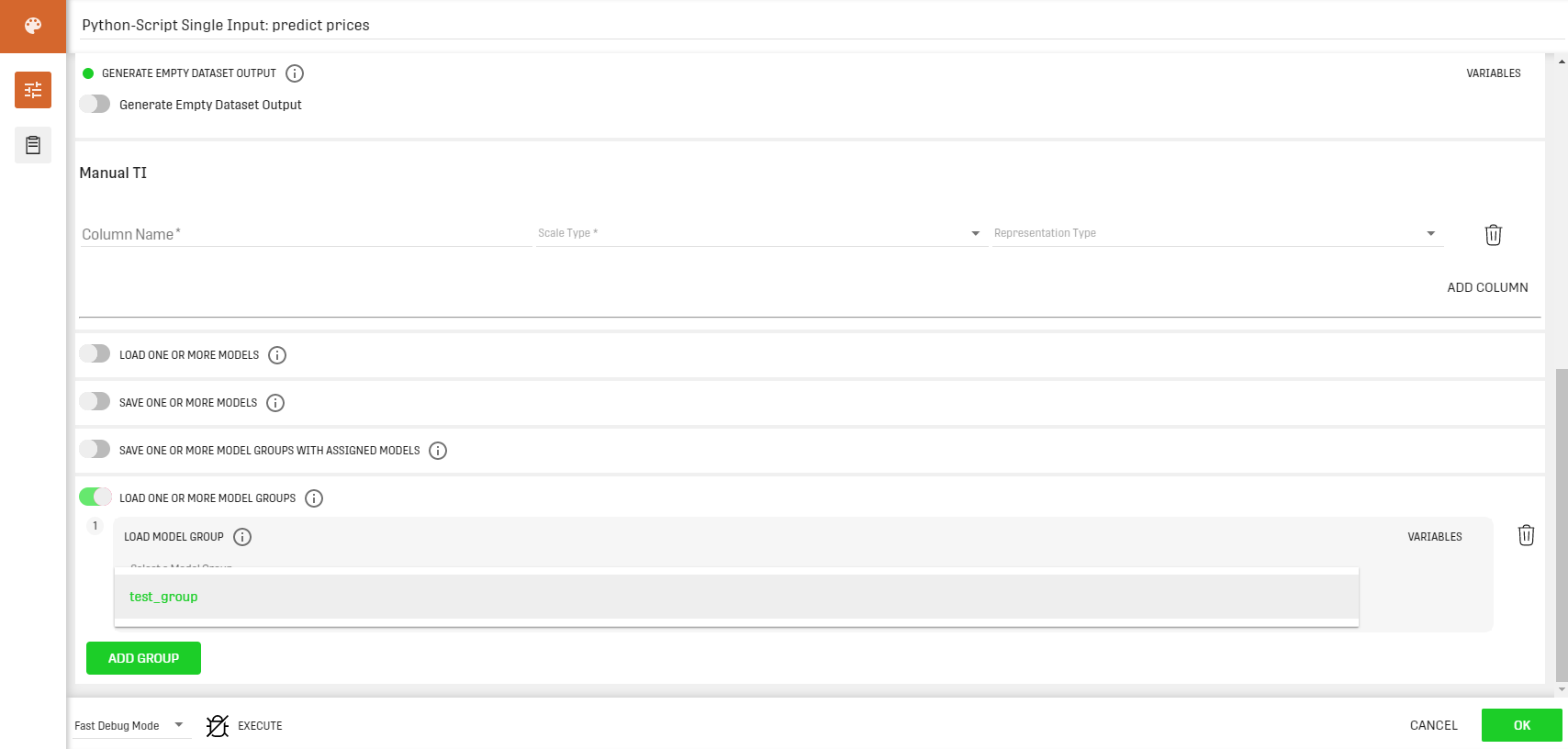# Example

In this example, we want to predict house prices for specific regions, according to Models we dynamically created and trained with sample data. For that, we will need two Workflows, one for creating the Models, one for predicting the prices.

The next sections will explain them in detail.

(The used datasets and the Workflows are attached at the bottom of the article.)

## Workflow for Creating the Models

First of all, we will load a dataset containing the training data for the Models. It contains regions with area codes and respective house prices. This is a snippet of the first 10 rows of the dataset: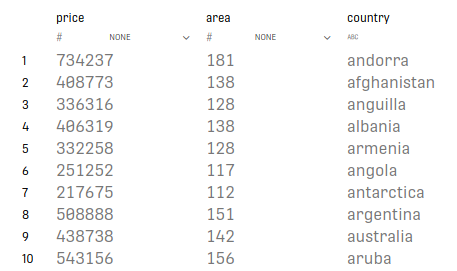It is loaded with a Data Table Load Processor, and then processed by a Python Script Single Input Processor. At the end, we added a Result Table in we which will not see any relevant results. We just need it, to have a valid Workflow set up.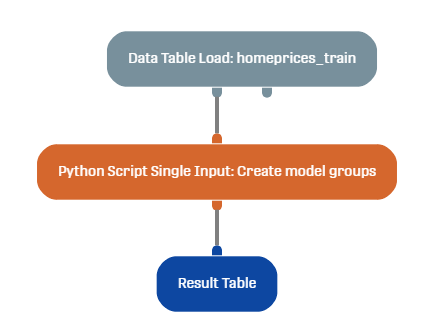Within the Processor configuration, we define a Python script that creates the Models and trains them by using the library sklearn. As output, we define the input dataset so the Processor returns a value. This is not mandatory, another option would be setting the "Generate Empty Dataset" option to true. This is the full script:

```from sklearn.linear_model import LinearRegression
import pandas as pd

df = od_input['input'].get_as_pandas()

# Select distinct countries
countries = df.country.unique()

# Count distinct countries
num_countries = len(countries)

# a matrix to write Models, number of Models = num of countries
models=[[]]*num_countries

# Go through all countries to create a Model
for idx in range(num_countries):
# Select rows for rows only for the current country
X_train = df[df['country'] == countries[idx]]
# Create Model name
model_name = countries[idx] + '_price_model'
models[idx]=LinearRegression()    # Train model
models[idx].fit(X_train[['area']], X_train['price'])

# publish your output: initial dataset

To save the created Models, the "Save One or More Model Groups" option in the Processor configuration is set to true. As name we need the exact same name mentioned in the Python script: "docu_example_predict_homeprices_group".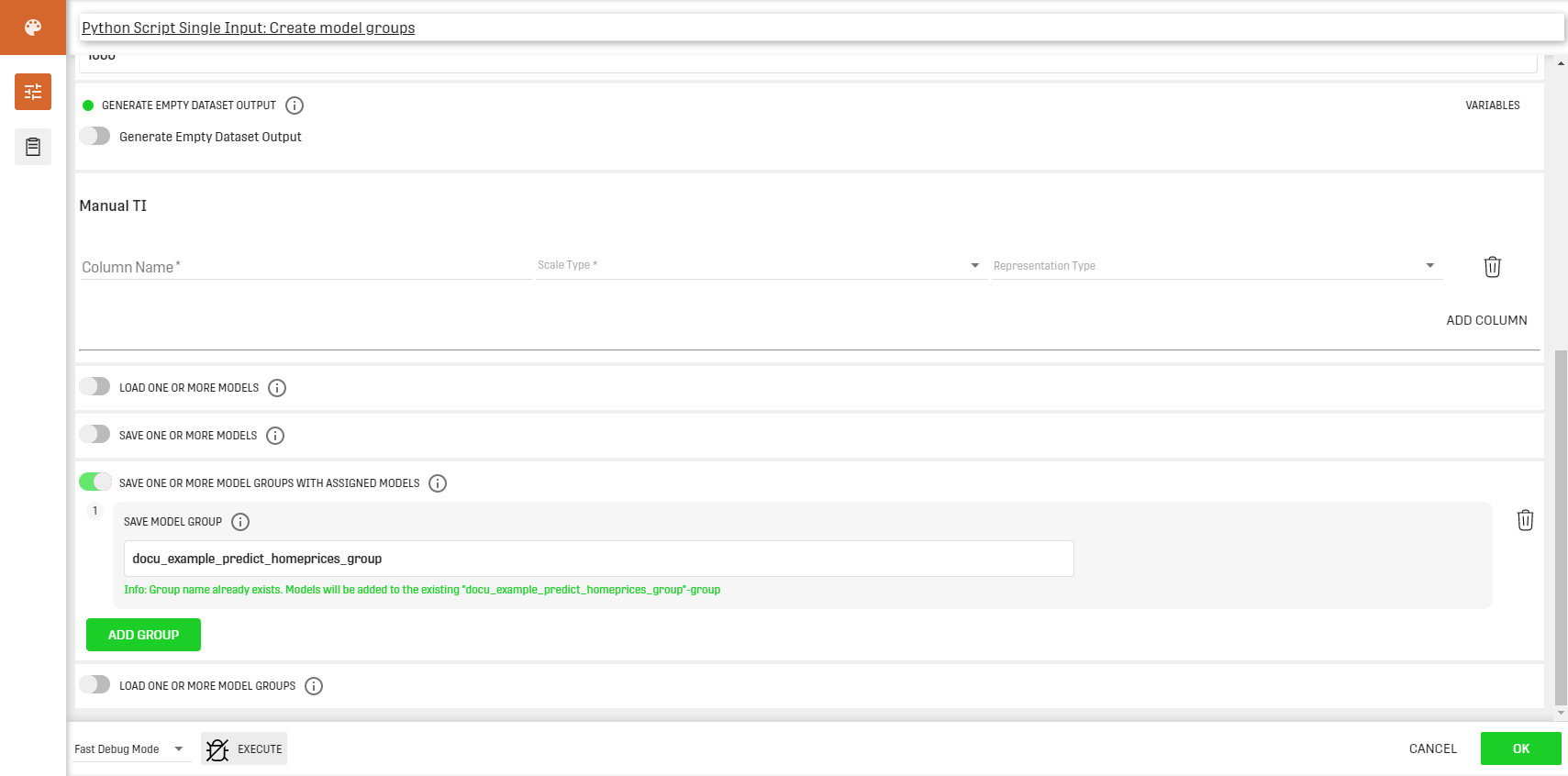### Result

After executing the Workflow, you should see that there is a new Model Group and 96 new Models available within your Domain/Project.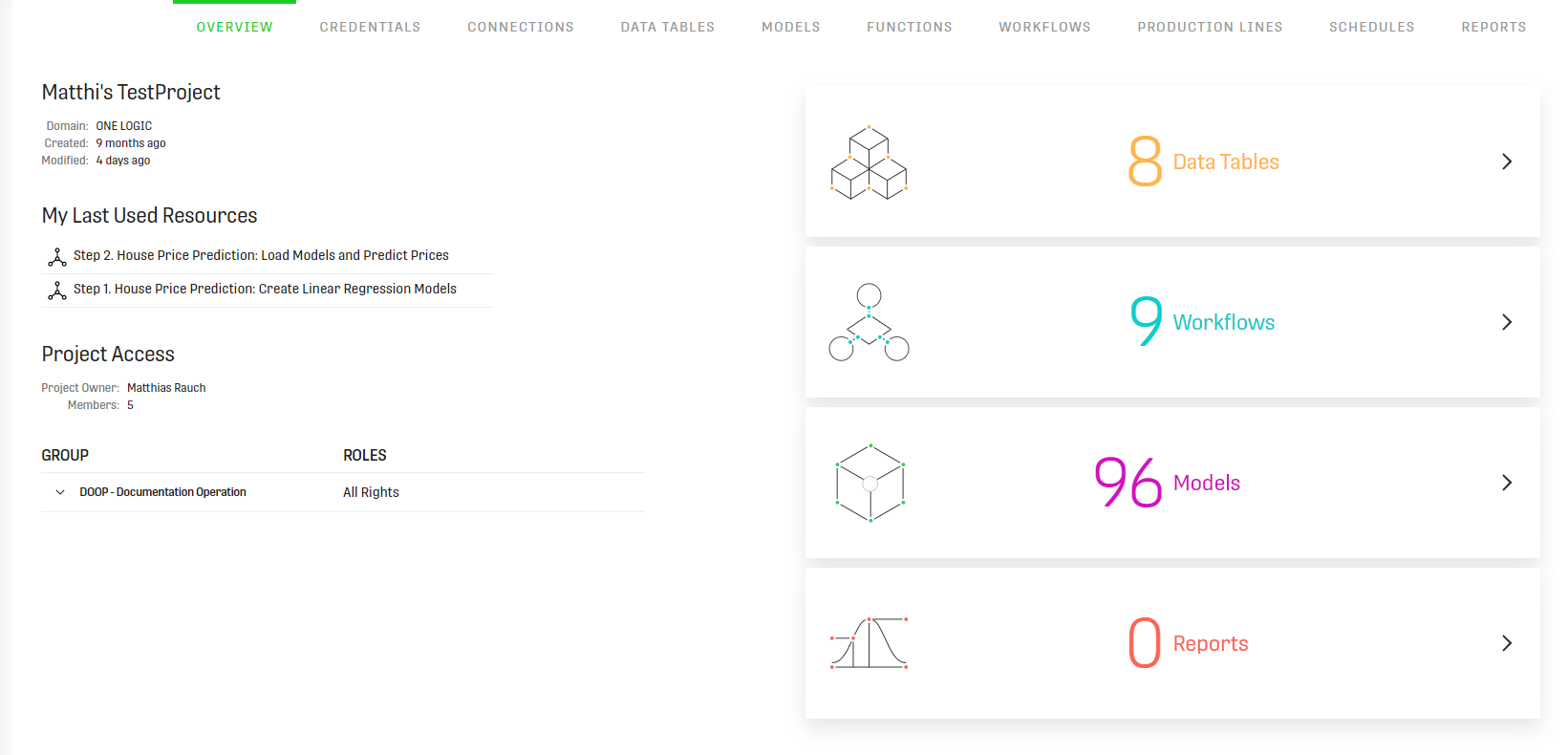## Workflow for Predicting the House Prices

Now that we have created our Models, we will use them to predict the house prices for the countries and areas listed in the second dataset. The schema is the following: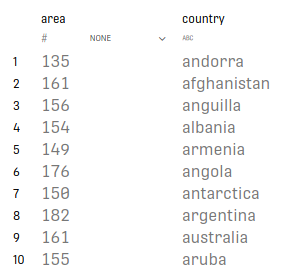It is also loaded with a Data Table Load Processor, and is then processed by a Python Script Single Input Processor. The final results are saved into a Result Table.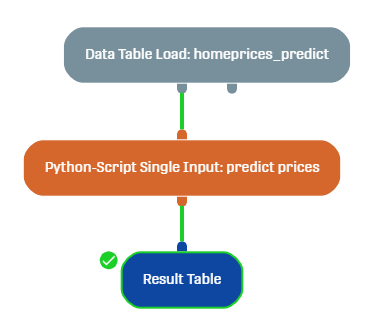Here we defined a script that iterates through all the rows of the input dataset and predicts the house prices for the given country and area. This is the code:

```import pandas as pd

pd.options.mode.chained_assignment = None

# od_input keys represent name of the input dataset set in OD Processor
df = od_input['input'].get_as_pandas()

# Select distinct countries
countries = df.country.unique()

# Count distinct countries
num_countries = len(countries)

# a matrix to write Models, number of Models = num of countries
models=[[]]*num_countries

#define empty output dataset
df_output = pd.DataFrame(columns = ['area', 'price', 'country'])

# Go through all countries to create prediction
for idx in range(num_countries):
model_name = countries[idx] + '_price_model'
X_test = df[df['country'] == countries[idx]]

# find model for the current country
models[idx]= od_models[model_name]
prediction = models[idx].predict(X_test[['area']])
X_test['price']=prediction
df_output = pd.concat([df_output, X_test])

#Output of predictions for every country

### Result

After execution of the Workflow, we can see the predicted house prices for the listed countries and areas in the input dataset: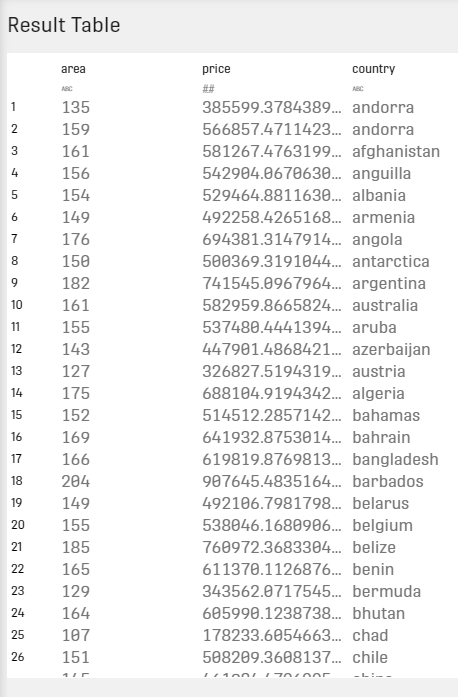# Related Articles

ONE DATA Python Framework

Python Script Data Generator Processor

Python Script Single Input Processor

Python Script Dual Input Processor

Models